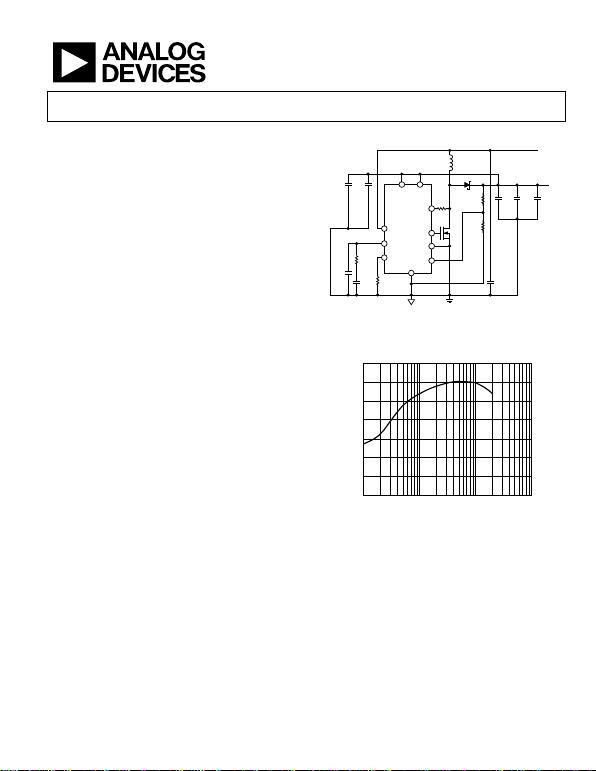Constant-Frequency, Current-Mode
Step-Up DC/DC Controller
Data Sheet
Rev. C Document Feedback
Information furnished by Analog Devices is believed to be accurate and reliable. However, no
responsibility is assumed by Analog Devices for its use, nor for any infringements of patents or other
rights of third parties that may result from its use. Specifications subject to change without notice. No
license is granted by implication or otherwise under any patent or patent rights of Analog Devices.
One Technology Way, P.O. Box 9106, Norwood, MA 02062-9106, U.S.A.
Technical Support www.analog.com
FEATURES
92% efficiency (no sense resistor required)
±1.0% initial accuracy
IC supply voltage range: 2.9 V to 5.5 V
Power-input voltage as low as 1.0 V
Capable of high supply input voltage (>5.5 V)
with an external NPN or a resistor
VIN UVLO and 35 mA shunt regulator
External slope compensation with 1 resistor
Programmable operating frequency
(100 kHz to 1.5 MHz) with 1 resistor
Lossless current sensing for switch-node voltage <30 V
Resistor current sensing for switch-node voltage >30 V
Synchronizable to external clock
Current-mode operation for excellent line and load transient
responses
10 µA shutdown current
Current limit and thermal overload protection
Soft start in 2048 clock cycles
APPLICATIONS
APD bias
Portable electronic equipment
Isolated dc/dc converter
Step-up/step-down dc/dc converter
LED driver for laptop computer and navigation system
LCD backlighting
GENERAL DESCRIPTION
The ADP1621 is a fixed-frequency, pulse-width modulation
(PWM), current-mode, step-up converter controller. It drives an
external n-channel MOSFET to convert the input voltage to a
higher output voltage. The ADP1621 can also be used to drive
flyback, SEPIC, and forward converter topologies, either isolated
or nonisolated.
The ADP1621 eliminates the use of a current-sense power
resistor by measuring the voltage drop across the on resistance of
the n-channel MOSFET. This technique, allowed up to a maximum
voltage of 30 V at the switch node, maximizes efficiency and
reduces cost. For switch-node voltages higher than 30 V or for
more accurate current limiting, the CS pin can be connected to a
current-sense resistor in the source of the MOSFET. The slope
compensation is implemented by an external resistor, allowing a
wide range of external components (inductors and MOSFETs),
and can be chosen for various switching frequencies and input
and output voltages.
TYPICAL APPLICATION CIRCUIT
IN
GATE
PGND
AGND
FB
SDSN
COMP
FREQ
GND
PIN
CS
R1
35.7kΩ
1%
R2
11.5kΩ
1%
C1
47µF
6.3V
L1
4.7µH
M1
C
COMP
1.8nF
C
OUT1
1µF
10V
f
OSC
= 600kHz
C1 = MURATA GRM31CR60J476M
C
OUT3
= SANYO POSCAP 6TPE150 M
L1 = TOKO FDV0630-4R7M
M1 = VI SHAY Si7882DP
D1 = VISHAY SSA33L
R
S
80Ω C
OUT2
10µF
10V
R
FREQ
31.6kΩ
1%
C
OUT3
150µF
6.3V
×2
V
OUT
= 5V
1A
V
IN
= 3.3V
D1
C3
1µF
10V
C4
0.1µF
10V
C2
120pF
R
COMP
9.09kΩ
06090-001
Figure 1. High Efficiency Output Boost Converter in Lossless Mode,
3.3 V Input, 5 V Output (Bootstrapped)
100
30
0.01 10
EFFI CIENCY ( %)
90
80
70
60
50
40
0.1 1
06090-042
Figure 2. Efficiency of Circuit Shown in Figure 1
The ADP1621 supply input voltage range is 2.9 V to 5.5 V,
although higher input voltages are possible with the use of a
small signal NPN pass transistor or a single resistor. The voltage
of the power input can be as low as 1 V for fuel cell applications.
The switching frequency is set by an external resistor over a range
of 100 kHz to 1.5 MHz and can be synchronized to an external
clock by using the SDSN pin. The shutdown quiescent current is
less than 10 µA. The ADP1621 has a thermal shutdown feature
that shuts down the gate driver when the junction temperature
reaches approximately 150°C. The internal soft start circuit limits
MSOP lead-free package and is specified over the −40°C to +125°C
junction temperature range.Rev. C | Page 2 of 32
Features .............................................................................................. 1
Applications ....................................................................................... 1
General Description ......................................................................... 1
Typical Application Circuit ............................................................. 1
Revision History ............................................................................... 2
Simplified Block Diagram ............................................................... 3
Specifications ..................................................................................... 4
Absolute Maximum Ratings ............................................................ 6
Thermal Resistance ...................................................................... 6
ESD Caution .................................................................................. 6
Pin Configuration and Function Descriptions ............................. 7
Typical Performance Characteristics ............................................. 8
Theory of Operation ...................................................................... 12
Control Loop ............................................................................... 12
Current-Sense Configurations .................................................. 12
Current Limit .............................................................................. 13
Undervoltage Lockout ............................................................... 13
Shutdown ..................................................................................... 13
Soft Start ...................................................................................... 13
Internal Shunt Regulators .......................................................... 13
Setting the Oscillator Frequency and Synchronization
Frequency .................................................................................... 13
Application Information: Boost Converter ................................. 14
Duty Cycle ................................................................................... 14
Setting the Output Voltage ........................................................ 14
Inductor Current Ripple ............................................................ 14
Inductor Selection ...................................................................... 14
Input Capacitor Selection .......................................................... 15
Output Capacitor Selection ....................................................... 15
Diode Selection ........................................................................... 16
MOSFET Selection ..................................................................... 16
Loop Compensation .................................................................. 17
Slope Compensation .................................................................. 18
Current Limit .............................................................................. 18
Recommended Component Manufacturers ........................... 19
Layout Considerations ................................................................... 20
Efficiency Considerations ............................................................. 21
Examples of Application Circuits ................................................. 22
Standard Boost ConverterDesign Example ........................ 22
Bootstrapped Boost Converter ................................................. 23
SEPIC Converter Circuit ........................................................... 27
Low Voltage Power-Input Circuit ............................................ 27
LED Driver Application Circuits ............................................. 28
Related Devices ............................................................................... 30
Outline Dimensions ....................................................................... 31
Ordering Guide .......................................................................... 31
REVISION HISTORY
10/15—Rev. B to Rev. C
Moved Figure 3 ................................................................................. 3
Changes to Shunt Regulation Voltage Parameter, Table 1 .......... 4
6/12—Rev. A to Rev. B
Change to Features Section ............................................................. 1
Change to Table 6 ........................................................................... 30
Updated Outline Dimensions ....................................................... 31
Changes to Ordering Guide .......................................................... 31
12/06—Rev. 0 to Rev. A
Changes to Table 1 ............................................................................. 3
Changes to Table 2 ............................................................................. 5
Changes to Table 5 .......................................................................... 19
Changes to Ordering Guide .......................................................... 31
7/06—Revision 0: Initial Version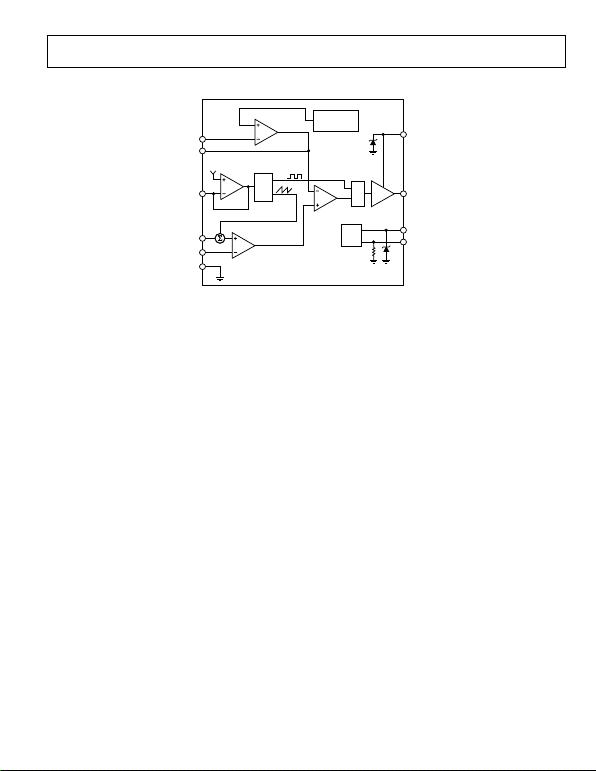Rev. C | Page 3 of 32
SIMPLIFIED BLOCK DIAGRAM
CS
PGND
FREQ OSC
VOSC
1.4V
VREF
1.215V
5.5V
5.5V
100kΩ
R
S
SET
SLOPE
COMP PWM
COMPARATOR
GATE
DRIVER
ERROR
AMPLIFIER
GND
FB
GATE
PIN
COMP
IN
SDSN
gm
n
+
+
SOFT START
(2048 CYCLES)
UVLO
06090-002
Figure 3. ADP1621 Simplified Block Diagram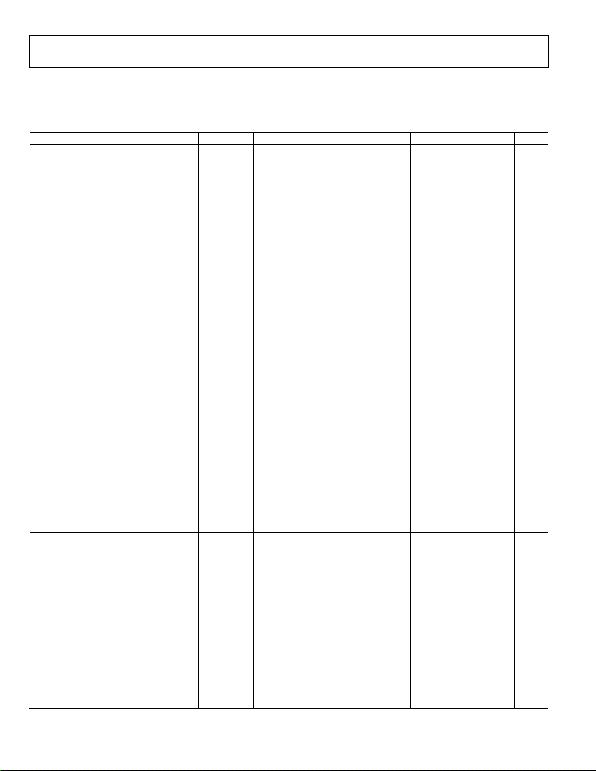Rev. C | Page 4 of 32
SPECIFICATIONS
VIN = 5 V, R FREQ = 100 kΩ, fOSC = 200 kHz, TJ = −40°C to 125°C, unless otherwise noted.
Table 1.
Parameter Symbol Test Conditions/Comments Min Typ Max Unit
MAIN CONTROL LOOP
Internal Soft Start Time tSS 2048 Cycles
PIN Supply Voltage1 VPIN 2.9 VSHUNT V
IN Supply Voltage1 VIN 2.9 VSHUNT V
Shunt Regulation Voltage VSHUNT IIN = 3 mA, IPIN = 3 mA, TA = 25°C 5.2 5.4 5.7 V
IIN = 3 mA, IPIN = 3 mA 5.0 5.4 6.0 V
Shunt Resistance
SHUNT
Current into IN = 8 mA to 12 mA
13
Current into PIN = 8 mA to 12 mA 7
IN Quiescent Current IIN VIN = 2.9 V to 5.5 V, VFB = 1.215 V 1.8 3 mA
IN Shutdown Current VIN = 2.9 V to 5.5 V, SDSN = GND 1 10 µA
PIN Supply Current IPIN
Static Mode, No Switching VFB = 1.3 V, VCOMP < VC O M P, Z C T , GATE = 0 V 1 10 µA
Shutdown Mode SDSN = GND 1 10 µA
Undervoltage Lockout Threshold at
IN Pin
VUVLO VUVLO rising 2.2 2.5 2.8 V
VUVLO hysteresis −80 mV
FB Regulation Voltage VFB TA = 25°C 1.203 1.215 1.227 V
1.197 1.215 1.233 V
FB Input Current IFB VFB = 1.215 V, TA = 25°C −75 +25 +75 nA
Line Regulation2 ∆VFB/∆VIN 2.9 V ≤ VIN 5 V, TJ = −40°C to +85°C 0.02 0.06 %/V
2.9 V ≤ VIN 5 V, TJ = −40°C to +125°C 0.02 0.072 %/V
Load Regulation3 ∆VFB/∆VCOMP VCOMP = 1.4 V to 1.5 V −1 −0.1 %
Error Amplifier Transconductance gm 300 µS
COMP Zero-Current Threshold VC O M P, Z C T 0.85 1.0 1.15 V
COMP Clamp High Voltage VCOMP,CLAMP TJ = −40°C to +85°C 1.9 2.0 2.1 V
TJ = −40°C to +125°C 1.9 2.0 2.2 V
Current-Sense Amplifier Gain n 7.5 9.5 11.5 V/V
Peak Slope-Compensation Current at
CS Pin4
ISC,PK VCS = 0 V to 100 mV maximum across RS
(GATE high)
55 70 85 µA
CS Pin Leakage Current ICS,LEAK VCS = 30 V (GATE low) 5 µA
Shutdown Time tSD SDSN pin from high to low or left floating 50 µs
Thermal Shutdown Threshold5 TTMSD 150 °C
Thermal Shutdown Hysteresis5 −10 °C
OSCILLATOR
Oscillator Frequency Range6 fOSC 100 1500 kHz
Oscillator Frequency fOSC RFREQ = 65 kΩ, TA = 25°C 255 325 395 kHz
Oscillator Frequency Tempco fOSC,TC ±0.06 %/°C
SDSN Input Level Threshold
SDSN,THRESH
V
IN
= V
PIN
= 5 V
1.5
1.7
1.9
V
SDSN Threshold Hysteresis −0.19 V
SDSN Internal Pull-Down Resistor RSDSN 100 kΩ
Synchronization Minimum Pulse Width tSYNC,MIN VSDSN = 0 V to VIN 45 100 ns
Synchronization Maximum Pulse Width tSYNC,MAX VSDSN = 0 V to VIN 0.8/fSYNC ns
Synchronization Frequency
SYNC
110
1800
kHz
GATE Minimum On Time tON,MIN VFB = 1.215 V, VCOMP = 1.0 V 180 215 ns
GATE Minimum Off Time tOFF,MIN VFB = 1.215 V, VCOMP = 2.0 V 190 230 ns
Maximum Duty Cycle6, 7 DMAX fSW = 200 kHz, RFREQ = 100 kΩ 93 97 %
Recommended Maximum
Synchronized Frequency Ratio6, 8
fSYNC/fOSC fOSC = 200 kHz, RFREQ = 100 kΩ, fSYNC = fSW 1.1 1.2 1.4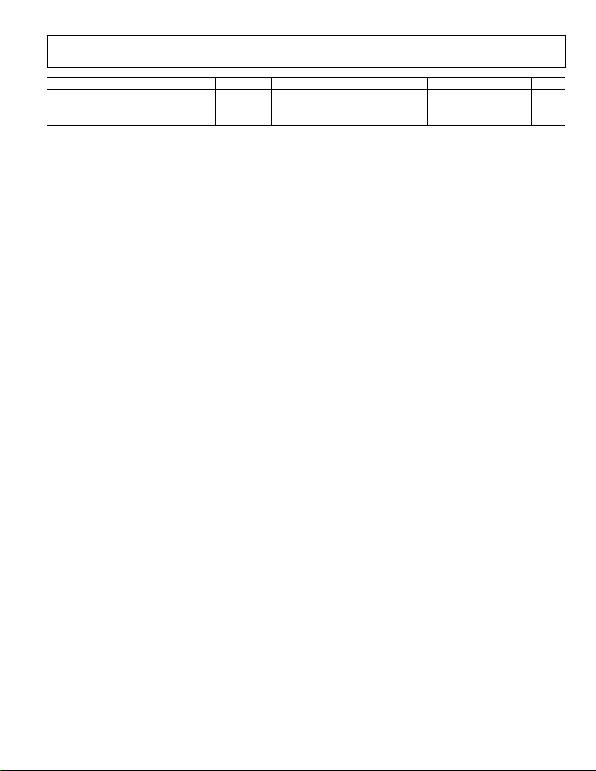Rev. C | Page 5 of 32
Parameter Symbol Test Conditions/Comments Min Typ Max Unit
GATE DRIVER
GATE Rise Time9 tR CGATE = 3.3 nF 17 ns
GATE Fall Time9 tF CGATE = 3.3 nF 13 ns
1 The maximum input voltage is the shunt regulation voltage, which is typically 5.5 V and can range from 5.3 V to 6.0 V over the specified temperature range.
2 The ADP1621 is tested in a feedback servo loop, which servos VFB to the internal reference voltage. The voltage change in FB is measured while VIN is changed from
2.9 V to 5 V. The line regulation is calculated by (∆VFB/VFB) × 100%/∆VIN.
3 The ADP1621 is tested in a feedback servo loop, which servos VFB to the internal reference voltage, and VCOMP is forced from 1.4 V to 1.5 V. The VCOMP range is
(1.0 V ≤ VCOMP ≤ 2.0 V).
4 The peak slope-compensation current at the CS pin is typically 70 µA, and effectively clamped at 116 mV. Thus, RS should not exceed 1.6 kΩ (116 mV/70 µA).
5 Guaranteed by design for thermal shutdown. When the thermal junction temperature of the ADP1621 reaches approximately 150°C, the ADP1621 goes into thermal
shutdown and the GATE voltage is pulled low. When the junction temperature drops below about 140°C, the soft start sequence is initiated and the ADP1621 resumes
normal operation.
6 fOSC is the natural oscillation frequency, fSYNC is the synchronization frequency, and fSW is the switching frequency. If synchronization is used, then fSW = fSYNC; otherwise, fSW = fOSC.
7 Guaranteed by design and bench characterization.
8 To ensure proper synchronization operation, set the synchronization frequency, fSYNC, to 1.2× of the free-running frequency, fOSC. Although the switching frequency can
be synchronized to as high as 1.8 MHz, the peak slope-compensation current decreases at higher synchronization frequencies. It is recommended that the maximum
fSYNC be less than 1.4× of fOSC and should not exceed 1.8 MHz. Choose the slope-compensation resistor, RS, for the synchronization frequency (see the Slope
Compensation section in the Application Information: Boost Converter section).
9 GATE rise and fall times are measured from 10% to 90% levels.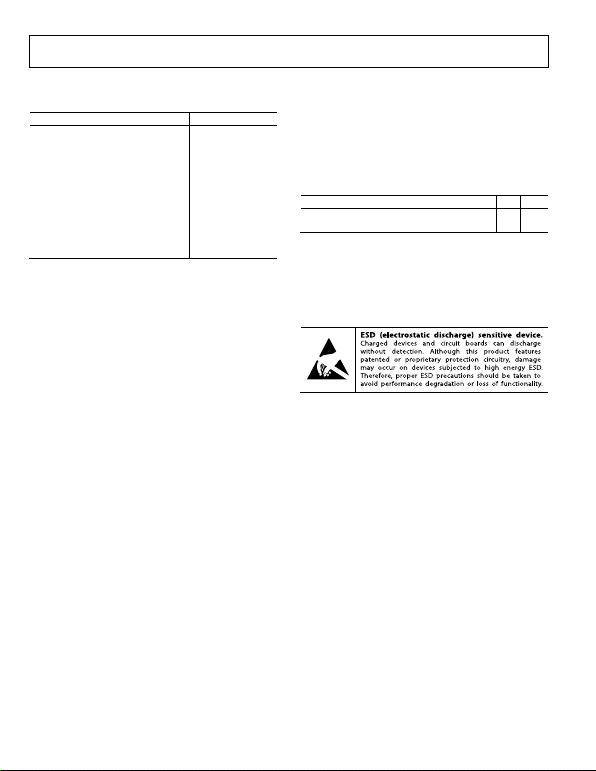Rev. C | Page 6 of 32
ABSOLUTE MAXIMUM RATINGS
Table 2.
Parameter Rating
IN to GND 0.3 V to VSHUNT
FB, COMP, SDSN, FREQ, GATE to GND
−0.3 V to (V
IN
+ 0.3 V)
CS to GND −5 V to +33 V
PIN to PGND −0.3 V to VSHUNT
Supply Current into IN 25 mA
Supply Current into PIN 35 mA
Storage Temperature Range −55°C to +150°C
Junction Operating Temperature Range1 −55°C to +150°C
Junction Storage Temperature Range −55°C to +150°C
Lead Temperature (Soldering, 10 sec) 300°C
Package Power Dissipation1 (TJ,MAX − TA)/θJA
1 In applications where high power dissipation and poor package thermal
resistance are present, the maximum ambient temperature may need to be
derated. Maximum ambient temperature (TA,MAX) is dependent on the maximum
operating junction temperature (TJ,MAX = 150oC), the maximum power dissipation
of the device in the application (PD,MAX), and the junction-to-ambient thermal
resistance of the package in the application (θJA), is given by the following
equation: TA,MAX = TJ,MAX JA x PD,MAX).
Stresses at or above those listed under Absolute Maximum
Ratings may cause permanent damage to the product. This is a
stress rating only; functional operation of the product at these
or any other conditions above those indicated in the operational
section of this specification is not implied. Operation beyond
the maximum operating conditions for extended periods may
affect product reliability.
Absolute maximum ratings apply individually only, not in
combination. Unless otherwise specified, all other voltages are
referenced to GND.
THERMAL RESISTANCE
θJA is specified for the worst-case conditions, that is, a device
soldered in a circuit board for surface-mount packages.
Table 3. Thermal Resistance
Package Type θJA Unit
10-Lead MSOP on a 2-Layer Printed Circuit Board (PCB) 200 °C/W
10-Lead MSOP on a 4-Layer PCB 172 °C/W
Junction-to-ambient thermal resistance of the package is based
on modeling and calculation using 2-layer and 4-layer boards, and
natural convection. The junction-to-ambient thermal resistance is
application- and board-layout dependent. In applications where
high maximum power dissipation exists, attention to thermal
dissipation issues in board design is required.
ESD CAUTION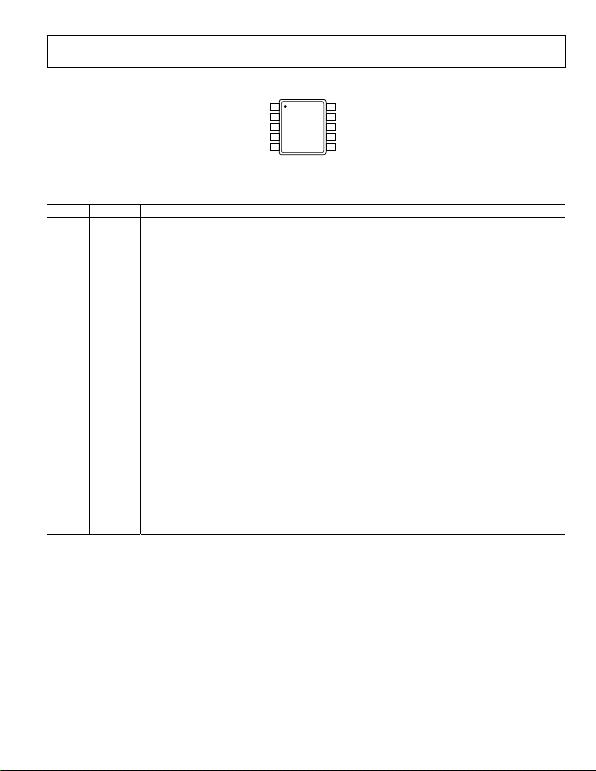Rev. C | Page 7 of 32
PIN CONFIGURATION AND FUNCTION DESCRIPTIONS
SDSN 1
GND 2
C
OMP 3
FB 4
FREQ 5
IN10
CS9
PIN
8
GATE7
PGND6
TOP VIEW
(Not to Scal e)
06090-003
Figure 4. Pin Configuration
Table 4. Pin Function Descriptions
Pin No. Mnemonic Description
1 SDSN
Shutdown and Synchronization Input. Turn the ADP1621 on by driving SDSN high; turn it off by driving SDSN low.
If SDSN is left floating or when the SDSN is pulled low, the ADP1621 goes into shutdown after 50 μs. If synchronization is
needed, synchronize the switching frequency to an external clock by connecting the external clock to the SDSN pin. An
internal 100 kΩ pull-down resistor is connected from SDSN to GND.
2 GND Ground.
3 COMP
Regulation Control Compensation Node. COMP is the output of the internal transconductance error amplifier.
Connect a series RC from COMP to GND to compensate the regulator. The nominal voltage range for this pin is
1.0 V to 2.0 V.
4 FB Feedback Input. FB is the input to the internal transconductance error amplifier. Drive FB from the output voltage
through a resistive voltage divider. The ratio of the voltage divider sets the output voltage. The regulation voltage
at FB is nominally 1.215 V.
5 FREQ
Frequency Control Input. Connect a resistor from FREQ to GND to set the free-running switching frequency
between 100 kHz and 1.5 MHz. The nominal voltage of this pin is 1.4 V.
6 PGND
Power Ground Input. PGND is the ground return for the internal gate driver and the negative input of the internal
current-sense amplifier. Connect PGND to GND as close to the ADP1621 as possible.
7 GATE
Gate Driver Output. The maximum gate driver output is equal to the PIN voltage. GATE drives the gate of the
external n-channel power MOSFET. Connect GATE to the gate of the MOSFET.
8 PIN Power Input. PIN powers the gate driver output. An internal 5.5 V shunt regulator is connected to this pin. Bypass
PIN to PGND with a 0.1 μF or greater capacitor.
9 CS Current-Sense Input. CS is the positive input of the current-sense amplifier. When GATE is turned on, the voltage at
the CS pin increases linearly from 0 V to a maximum of 116 mV, and the nominal peak slope-compensation output
current is 70 μA. When GATE is off, the CS function is disabled. For current sensing in lossless mode, connect CS to
the drain of the power MOSFET. The absolute maximum voltage at CS is 33 V. For higher accuracy current sensing
or higher switch-node voltages, connect CS to a current-sense power resistor in the source of the power MOSFET.
In both sensing methods, it is required to add a slope-compensation resistor, RS, to the CS pin to achieve stability
in the inductor current for duty cycles greater than 50%. However, it is recommended to add RS for all duty cycles
because load transients can momentarily cause the duty cycle to be greater than 50%, even when the steady-
state duty cycle is less than 50%.
10 IN Input Voltage. IN powers the ADP1621 internal circuitry. An internal 5.5 V shunt regulator is connected to this pin.
Bypass IN to GND with a 0.1 μF or greater capacitor.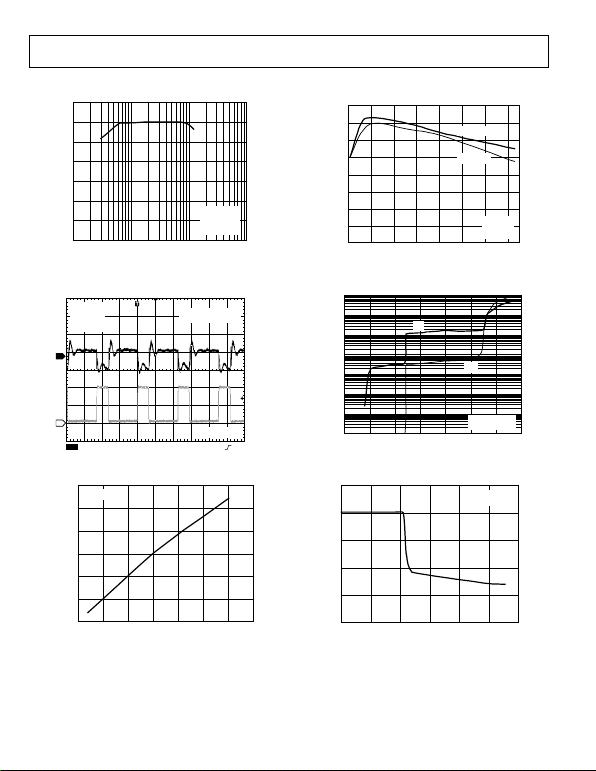Rev. C | Page 8 of 32
TYPICAL PERFORMANCE CHARACTERISTICS
100
30
0.01 10
LO A D CU RRENT (A)
EFFICIENCY (%)
90
80
70
60
50
40
0.1 1
T
A
= 25°C
f
SW
= 220kHz
V
IN
= 3.3V
V
OUT
= 5V
06090-004
Figure 5. Efficiency vs. Load Current
CH1 20mV CH2 2V M2µs A CH2 2.6V
1
2
T
A
= 25° C
V
IN
= 3.3V
V
OUT
= 5V
V
OUT
RIPPLES @ 5V
AC-COUPLED
CH2 = G ATE
0
6090-005
Figure 6. Output Voltage Ripple of the Circuit Shown in Figure 1
1.21605
1.215752.5 6.0
VIN (V)
VFB (V)
TA=25°C
1.21600
1.21595
1.21590
1.21585
1.21580
3.0 3.5 4.0 4.5 5.0 5.5
06090-006
Figure 7. VFB vs. VIN
92
84
100
SWI TCHING FREQUENCY (kHz)
EFFICIENCY (%)
91
90
89
88
87
86
85
300 500 700 900 1100 1300 1500
T
A
= 25°C
V
IN
= 3.3V
V
OUT
= 5V
06090-007
Figure 8. Efficiency vs. Switching Frequency
100
0.00001 07
SUPPLY VOLTAGE (V)
SUPPL Y CURRENT (mA)
T
A
= 25°C
NO SWITCHING
10
1
0.1
0.01
0.001
0.0001
123456
I
IN
I
PIN
06090-008
Figure 9. Supply Current vs. Supply Voltage
2.5
0
1.17 1.29
V
FB
(V)
V
COMP
(V)
T
A
= 25°C
V
IN
= 5V
2.0
1.5
1.0
0.5
1.19 1.21 1.23 1.25 1.27
06090-009
Figure 10. VCOMP vs. VFB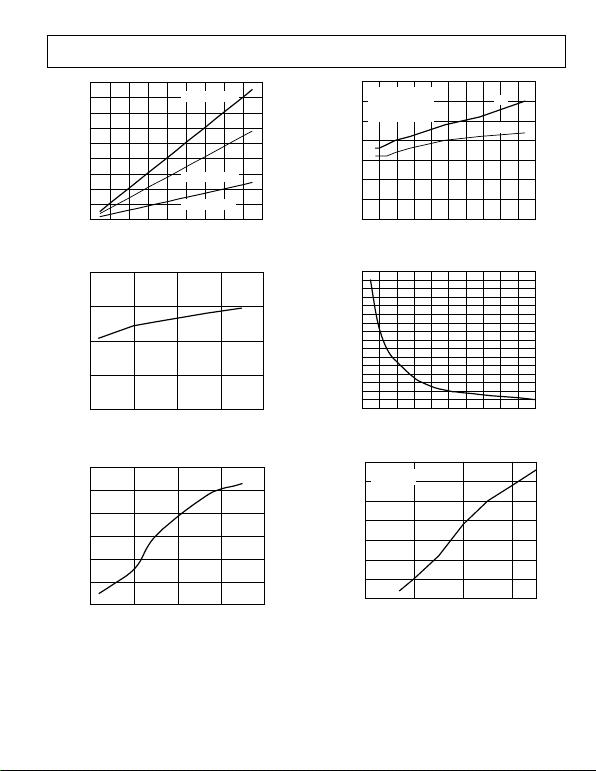Rev. C | Page 9 of 32
45
001800
SWIT CHING FREQ UE NCY ( kHz )
PIN S UP P LY CURRE NT (mA)
MOSFET QG = 25nC
MOSFET QG = 15nC
MOSFET QG = 7nC
40
35
30
25
20
15
10
5
200 400 600 800 1000 1200 1400 1600
06090-010
Figure 11. PIN Supply Current vs. Switching Frequency
2.60
2.40
–50 150
TEMPERATURE (°C)
V
UVLO
(V)
SDSN = 5V
2.55
2.50
2.45
050 100
06090-011
Figure 12. VUVLO Threshold vs. Temperature
1.03
0.97
–50 150
TEMPERATURE (°C)
NORMALI ZED FREQ UE NCY ( fOSC/fOSC,25°C)
050 100
1.02
1.01
1.00
0.99
0.98
VIN = 5V
06090-012
Figure 13. Frequency vs. Temperature
35
0050
GAT E CAP ACIT ANCE ( nF )
GATE RISE AND FALL TIMES (ns)
30
25
20
15
10
5
510 15 20 25 30 35 40 45
TA = 25° C
VIN = VPIN = 5V
tR OR tFIS FROM
10% TO 90% OF
THE GATE VOLTAGE tF
tR
06090-013
Figure 14. GATE Rise and Fall Times vs. CGATE
1600
00200
R
FREQ
(k)
fOSC
(kHz)
1500
1400
1300
1200
1100
1000
900
800
700
600
500
400
300
200
100
20 40 60 80 100 120 140 160 180
06090-014
Figure 15. Oscillator Frequency vs. Resistance
198
191 2
VIN (V)
fOSC (kHz)
197
196
195
194
193
192
3 4 5
TA = 25° C
RFREQ = 100k
06090-015
Figure 16. Oscillator Frequency vs. VIN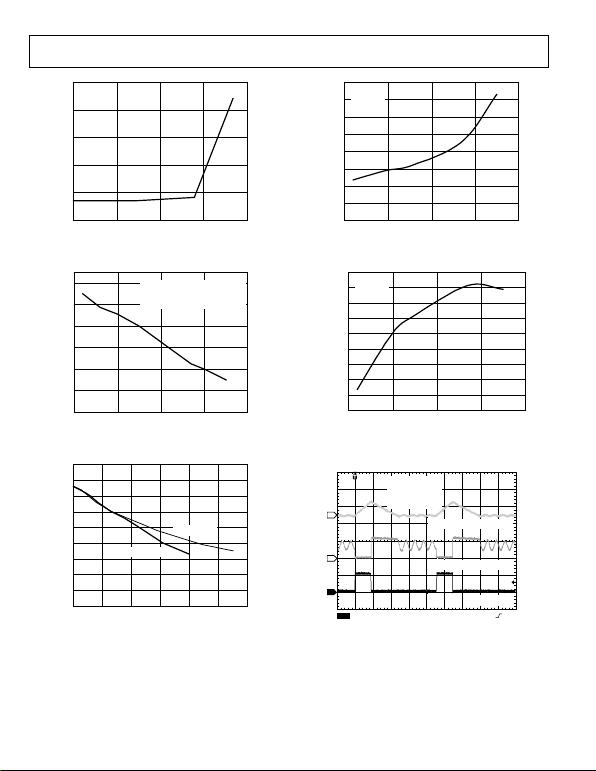Rev. C | Page 10 of 32
250
0
–40 160
CS LEAKAGE (nA)
TEMPERATURE (°C)
V
IN
= 5V
CS = 30V
200
150
100
50
10 60 110
06090-016
Figure 17. Temperature vs. CS Leakage
8
–16
–50 150
TEMPERATURE (°C)
FB BIAS CURRENT (nA)
4
0
–4
–8
–12
050100
VFB = 1.2113V AT 25°C
FB BIAS CURRENT IS MEASURED
BY FORCING A CONSTANT 1.2113V
OVER THE TEMPERATURE RANGE.
06090-017
Figure 18. FB Bias Current vs. Temperature
90
0
1.0
f
SYNC
/
f
OSC
PEAK SLOPE COMPENSATION CURRENT (µA)
2.2
f
OSC
= 200kHz
f
OSC
= 550kHz
80
70
60
50
40
30
20
10
1.2 1.4 1.6 1.8 2.0
06090-018
Figure 19. Slope-Compensation Current vs. fSYNC/fOSC
1.6
0
–50 150
TEMPERATUREC)
SHUTDOWN IN CURRENT (µA)
050100
1.4
1.2
1.0
0.8
0.6
0.4
0.2
V
IN
= 5V
SDSN = 0V
06090-019
Figure 20. Shutdown IN Current vs. Temperature
1.2165
1.2120
–50 150
TEMPERATURE (°C)
V
FB
(V)
050100
V
IN
= 5V
SDSN = 0V
1.2160
1.2155
1.2150
1.2145
1.2140
1.2135
1.2130
1.2125
06090-020
Figure 21. FB Voltage vs. Temperature
CH1 5V CH2 5V
CH4 500mA
M2µs A CH1 2.9V
1
2
4
T
A
= 25°C
V
IN
= 3.3V
V
OUT
= 5V
DCM OPERATION
CH1 = GATE
CH4 = INDUCTOR CURRENT
CH2 = DRAIN VOLTAGE
06090-021
Figure 22. DCM Switching Waveform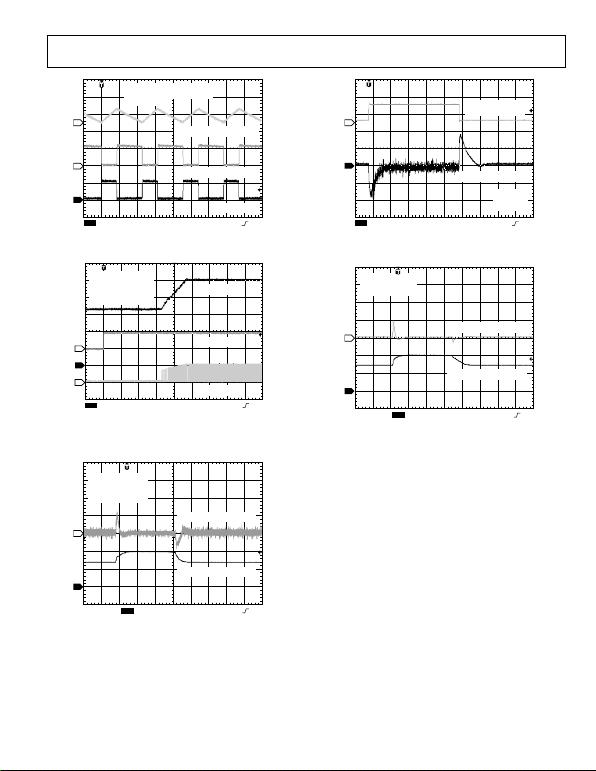Rev. C | Page 11 of 32
CH1 5V CH2 5V
CH4 500mA
M2µs A CH1 2.9V
1
2
4
T
A
= 25°C
V
IN
= 3.3V
V
OUT
= 5V
CCM OPERATION
CH1 = GATE
CH4 = INDUCTOR CURRENT
CH2 = DRAIN VOLTAGE
06090-022
Figure 23. CCM Switching Waveform
CH1 1V
CH3 5V
CH2 5V M2ms A CH1 4.5V
1
2
3
T
A
= 25°C
V
IN
= 3.3V
V
OUT
= 5V
f
SW
= 220kHz
SOFT-START = 9.3ms
CH3 = GATE
CH1 = V
OUT
CH2 = SDSN
06090-023
Figure 24. Soft Start Waveform
CH1 50mV CH2 2V M400µs A CH2 3.8V
2
1
T
A
= 25°C
V
OUT
= 5V
OUT
= 1A
CH1 = V
OUT
, AC-COUPLED
CH2 = V
IN
FROM 3V TO 4V
0
6090-024
Figure 25. Line Transient Response of the Configuration Shown in Figure 1
CH1 50mV
CH4 1A
M200µs A CH4 700V
1
4
T
A
= 25°C
V
IN
= 3.3V
V
OUT
= 5V
FROM 0.2A TO 1.2A
OUTPUT, AC-COUPLED
06090-025
Figure 26. Load Transient Response of the Circuit Shown in Figure 1
CH1 50mV CH2 2V M400µs A CH2 3.8V
2
1
T
A
= 25°C
V
OUT
= 5V
OUT
CH1 = V
OUT
, AC-COUPLED
CH2 = V
IN
FROM 3V TO 4V
0
6090-026
Figure 27. Line Transient Response of the Configuration Shown in Figure 1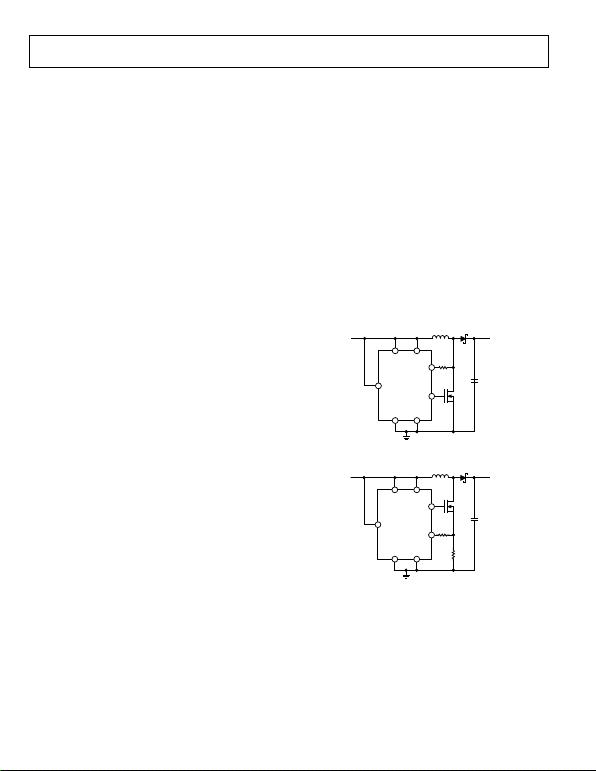Rev. C | Page 12 of 32
THEORY OF OPERATION
The ADP1621 is a fixed-frequency, current-mode, step-up dc/dc
converter controller. It drives an external n-channel MOSFET
to step the input voltage up to a higher output voltage. It can be
used for SEPIC, flyback, boost, buck-boost, forward, and other
converter topologies. It operates at a fixed switching frequency that
is set by an external resistor over a range of 100 kHz to 1.5 MHz,
and it can be synchronized to an external clock by connecting
the SDSN pin to the clock.
The input supply current to the ADP1621 is less than 3 mA
during normal operation and less than 10 µA during shutdown.
The ADP1621 can drive large external MOSFETs, allowing it to
support load currents in excess of 10 A.
CONTROL LOOP
The ADP1621 uses a current-mode architecture to regulate the
output voltage. The output voltage is monitored at FB through
a resistive voltage divider. The voltage at FB is compared to the
internal 1.215 V reference voltage by the internal transconductance
error amplifier to create an error current at COMP. A resistor-
capacitor compensation impedance connected from COMP to
GND converts the error current to an error voltage.
At the beginning of the switching cycle, the MOSFET is turned
on and the inductor current ramps up. The MOSFET current is
measured and converted to a voltage using RCS or RDSON and is
added to the stabilizing slope-compensation ramp. The resulting
voltage sum passes through the current-sense amplifier to generate
the current-sense voltage. When the current-sense voltage is
greater than the COMP error voltage, the MOSFET is turned off
and the inductor current ramps down until the internal clock
initiates the next switching cycle. The duty-cycle of the PWM
at the desired output voltage. Because the output voltage ultimately
controls the peak inductor current through the COMP error
voltage, this scheme is referred to as peak current-mode control.
With light loads, the converter can also operate under discon-
tinuous conduction mode and pulse-skipping modulation to
maintain output-voltage regulation. These two forms of operation
are discussed in detail in the Light Load Operation section.
Note that the converter can also be designed to operate in
discontinuous conduction mode at full load if desired.
Overall, the current-mode regulation system of the ADP1621
allows fast transient responses while maintaining a stable output
voltage. By selecting the proper resistor-capacitor network from
COMP to GND, the regulator response can be optimized for a
wide range of input voltages, output voltages, and load currents.
CURRENT-SENSE CONFIGURATIONS
The ADP1621 can sense the current across the on resistance of
the MOSFET to minimize external component count and improve
efficiency by eliminating the power that would be lost in a current-
sense resistor. This lossless technique eliminates the need for an
expensive current-sense resistor. In the lossless mode configuration,
the voltage at the CS pin (or the switch-node voltage at the drain of
the MOSFET) must not exceed 30 V (see Figure 28). This technique
maximizes efficiency and reduces cost. In practice, when the
calculated VSW approaches 30 V, build the board and measure
the actual VSW before committing to the lossless mode design.
Because of the parasitic inductance in the diode, output capacitor,
and PCB traces, VSW typically has narrow peaks that exceed the
theoretical maximum voltage at VSWthe sum of VOUT and the
forward-voltage drop of Diode D1. If the measured peak voltage
exceeds 30 V, or if a more accurate current limit is desired, then
the CS pin can be connected to an external current-sense resistor
in the source of the MOSFET (Figure 29). The maximum power
output is limited by the selection of the external components.
IN
GATE
SDSN
GND
PIN
CS
PGND
L
R
S
D1
V
IN
V
OUT
V
SW
C
O
06090-027
Figure 28. CS Pin Connection for VSW < 30 V, Lossless Mode
(No Current-Sense Resistor Needed)
IN
CS
SDSN
GND
PIN
GATE
PGND
L
R
S
D1
V
IN
V
OUT
R
CS
V
SW
C
O
06090-028
Figure 29. CS Pin Connection for VSW > 30 V, Resistor Sense Mode
with a Current-Sense Resistor, RCS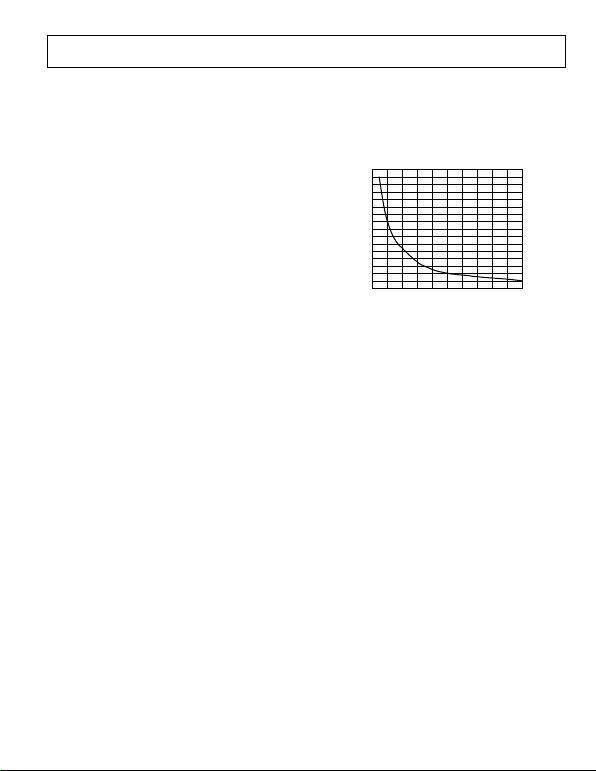Rev. C | Page 13 of 32
CURRENT LIMIT
The current limit is achieved by the COMP voltage clamp, owing
to the current-mode operation of the ADP1621. A detailed
explanation of how the current limit is determined can be found
in the Current Limit section of the Application Information:
Boost Converter section.
UNDERVOLTAGE LOCKOUT
An internal undervoltage lockout (UVLO) circuit at the IN pin
holds the GATE voltage low when the IN voltage is below the
UVLO voltage, which is typically 2.5 V.
SHUTDOWN
The ADP1621 goes into shutdown approximately 50 µs after the
SDSN pin is pulled low or left floating. There is an internal 100 kΩ
resistor connected between SDSN and GND.
150°C, the ADP1621 goes into thermal shutdown and the GATE
voltage is pulled low. When the junction temperature drops below
soft start sequence.
SOFT START
The ADP1621 has an internal soft start circuit that ramps
the FB regulation voltage from 0 V to 1.215 V in 64 steps over
2048 clock oscillator cycles. This soft start ramp allows the
output voltage to slowly rise to the steady-state output voltage,
preventing input inrush current at startup.
INTERNAL SHUNT REGULATORS
The IN and PIN pins each have an internal shunt regulator that
allows the ADP1621 to operate over a wide input voltage range.
The shunt regulators limit the voltages at IN and PIN to about
5.5 V, allowing the use of logic-level MOSFETs independent of
the input and/or output voltage. The shunt regulator voltage can
reach 5.7 V at 10 mA. See Figure 9 for the I-V characteristics of
these shunt regulators.
The internal power is derived from the IN pin, whereas the
MOSFET gate driver (GATE) current comes from the power
input, PIN. By separating the two inputs, PIN can be driven
with an external small-signal NPN transistor to limit the power
loss in the PIN shunt regulator when the input voltage is higher
than 5.5 V. See Figure 37 for an example. The maximum currents
going into PIN and IN must not exceed 35 mA and 25 mA,
respectively.
SETTING THE OSCILLATOR FREQUENCY AND
SYNCHRONIZATION FREQUENCY
The free-running oscillator frequency, fOSC, is set by a resistor
from FREQ to GND. A 100 kΩ resistor sets the typical oscillator
frequency to 200 kHz, a 65 kΩ resistor sets it to 325 kHz, a 32 kΩ
resistor sets it to 600 kHz, and a 10 kΩ resistor sets it to 1.5 MHz.
Figure 30 shows a typical relationship between fOSC and RFREQ.
1600
00200
R
FREQ
(k)
fOSC
(kHz)
1500
1400
1300
1200
1100
1000
900
800
700
600
500
400
300
200
100
20 40 60 80 100 120 140 160 180
06090-029
Figure 30. fOSC vs. RFREQ
The switching frequency can be synchronized to an external clock
by driving the SDSN pin with that clock signal. The SDSN pin
serves the two functions of shutdown control and frequency
synchronization input. If the SDSN input detects a low-to-high
transition within 10 µs of a high-to-low transition, it resets the
oscillator to synchronize to the frequency of the signal at SDSN.
The ADP1621 only synchronizes to frequencies greater than the
free-running switching frequency. To ensure proper synchronization
operation, set the synchronization frequency, fSYNC, to 1.2× the free-
running frequency, fOSC. The switching frequency, fSW, is equal to
fSYNC. Although the switching frequency can be synchronized to as
high as 1.8 MHz, the peak slope-compensation current decreases at
higher fSYNC. It is recommended that the maximum fSYNC be less than
1.4× of fOSC. Choose the slope-compensation resistor, RS, for the
synchronization frequency (see the Slope Compensation section).
For SDSN to detect a high input, the high state must remain
high for at least 100 ns.Rev. C | Page 14 of 32
APPLICATION INFORMATION: BOOST CONVERTER
In this section, an analysis of a boost converter is presented,
along with guidelines for component selection. A typical boost-
converter application circuit is shown in Figure 1.
ADIsimPower is a collection of tools that produce complete
power designs optimized for a specific design goal. The tools
enable the user to generate a full schematic, bill of materials,
and calculate performance in minutes. ADIsimPower can
optimize designs for cost, area, efficiency, and device count
while taking into consideration the operating conditions and
limitations of the IC and all real external components. For
www.analog.com/ADIsimPower. The tool set is available from
this website, and users can also request an unpopulated board
through the tool.
DUTY CYCLE
To determine the worst-case inductor current ripple, output voltage
ripple, and slope-compensation factor, it is first necessary to
determine the system duty cycle. The duty cycle in continuous
conduction mode (CCM) is calculated by the following equation:
D
OUT
IND
OUT
VV
VVV
D+
+
=
(1)
where:
VOUT is the desired output voltage.
VIN is the input voltage.
VD is the forward-voltage drop of the diode. A typical Schottky
diode has a forward-voltage drop of 0.5 V.
The GATE minimum on and off times determine the minimum
and maximum duty cycles, respectively. The minimum on and off
times are typically 180 ns and 190 ns, respectively. The minimum
and maximum duty cycles are given by the following equations:
SW
MINON
SW
MINON
MIN ft
t
t
D×== ,
,
(2)
)(11
,
,
SWMINOFF
SW
MINOFF
MAX
ft
t
t
D×==
(3)
where:
DMIN is the minimum duty cycle.
DMAX is the maximum duty cycle.
tON,MIN is the minimum on time.
tOF F, M I N is the minimum off time.
tSW is the switching period.
fSW is the switching frequency.
Note that when the converter tries to operate at a duty cycle
lower than DMIN, pulse-skipping modulation occurs to maintain the
output voltage regulation (see the Light Load Operation section).
SETTING THE OUTPUT VOLTAGE
The output voltage is set through a voltage divider from the output
voltage to the FB input. The feedback resistor ratio sets the output
voltage of the system. The regulation voltage at FB is 1.215 V. The
output voltage is given by the following equation (see Figure 1):
+×= R2
R1
V
OUT
1V215.1
(4)
The input bias current into FB is 25 nA typical, 70 nA maximum.
For a 0.1% degradation in regulation voltage and with 70 nA
bias current, R2 must be less than 18 kΩ, which results in 68 µA
of divider current. Choose the value of R1 to set the output
voltage. Using higher values for R2 results in reduced output
voltage accuracy due to the input bias current at the FB pin,
whereas lower values cause increased quiescent current
consumption.
INDUCTOR CURRENT RIPPLE
Choose a peak-to-peak inductor ripple current between 20%
and 40% of the average inductor current. A good starting point
for a design is to choose the peak-to-peak ripple current to be
30% of 1/(1 − D) times the maximum load current:
D
I
I
L
×= 1
3.0
,
(5)
where:
ΔIL is the peak-to-peak inductor ripple current.
INDUCTOR SELECTION
The inductor value choice is important because it dictates
the inductor current ripple and therefore the voltage ripple
at the output.
The average inductor current, IL,AVE, is given by the following
equation:
D
I
AVEL
=1
,
(6)
and the peak-to-peak inductor ripple current is inversely
proportional to the inductor value:
Lf
DV
I
SW
IN
L
×
×
=
(7)
where:
fSW is the switching frequency.
L is the inductor value.
Assuming continuous conduction mode (CCM) operation, the
peak inductor current is given by the following equation:
Lf
DV
D
I
I
D
I
I
SW
L
PKL
××
×
+
=
+
=2121
,
(8)Rev. C | Page 15 of 32
Smaller inductor values are typically smaller in size and usually
less expensive, but increase the ripple current. Larger ripple current
also increases the power loss in the inductor core. Too large an
transient responses because it reduces the effect of slope
compensation.
Assuming the ripple current is 30% of 1/(1 − D) times the max-
imum load current, a reasonable choice for the inductor value is
( )
IN
If
DDV
L
,
3.0
1
××
××
=
(9)
From this starting point, modify the inductance to obtain the
right balance of size, cost, and output voltage ripple while
maintaining the inductor ripple current between 20% and 40%
of 1/(1 − D) times the maximum load current. Keep in mind
that the inductor saturation current must be greater than the
peak inductor current. Magnetically shielded inductors are
generally recommended, although they cost slightly more than
unshielded inductors.
Also, losses due to the inductor winding resistance reduce the
efficiency of the boost converter. This power loss is given by
W
WL
R
D
I
P×
=
2
,
1
(10)
where:
PL,W is the power dissipation in the winding of the inductor.
RW is the winding resistance.
INPUT CAPACITOR SELECTION
The bulk input capacitor provides a low impedance path for the
inductor ripple current. Capacitor C1 in Figure 1 represents a
bulk input capacitor. Choose a bulk input capacitor whose
impedance at the switching frequency is lower than the
impedance of the voltage source VIN.
The preferred bulk input capacitor is a 10 µF to 100 µF ceramic
capacitor because it has low equivalent series resistance (ESR) and
low impedance. Aluminum electrolytic and aluminum polymer
capacitors can also be used as the bulk input capacitors. The bulk
input capacitor does not need to be placed very close to the IN
and PIN pins. Aluminum electrolytic capacitors are the cheapest
and generally have high ESR values, which increase dramatically at
temperatures less than 0°C. Some aluminum electrolytic capacitors
have ESR less than 20 mΩ, but their capacitances are generally
greater than 800 µF. Aluminum polymer capacitors are more
expensive than the aluminum electrolytic ones, but are generally
cheaper than the ceramic capacitors for the same amount of
capacitance. Polymer capacitors have relatively low ESR, with
some models having less than 10 mΩ.
Regardless of the type of capacitor used, make sure the ripple
current rating of the bulk input capacitor, ICIN,RMS, is greater than
2
3
1
,
L
RMSCIN
I
I
×=
(11)
where ΔIL is the peak-to-peak inductor ripple current.
In addition to the bulk input capacitor, a bypass input capacitor is
required. The function of the bypass capacitor is to locally filter the
input voltage to the ADP1621 and maintain the input voltage at a
steady value during switching transitions. The bypass capacitor is
typically a 0.1 µF or greater ceramic capacitor and should be placed
as close as possible to the IN and PIN pins of the ADP1621.
Capacitors C3 and C4 in Figure 1 represent the bypass capacitors.
OUTPUT CAPACITOR SELECTION
The output capacitor maintains the output voltage and supplies
current to the load while the external MOSFET is on.
The value and characteristics of the output capacitor greatly
affect the output voltage ripple and stability of the converter.
The amount of peak-to-peak output voltage ripple, ΔVOUT, can
be approximated by
×
+
21
L
OUT
I
D
I
V
( )
22
2
2
2
1ESL
fESR
Cf SW
OUT
SW
××π++
××π
(12)
where:
ΔIL is the peak-to-peak inductor ripple current.
fSW is the switching frequency.
COUT is the output capacitance.
ESR is the effective ESR of COUT.
ESL is the effective equivalent series inductance of COUT.
Because the output capacitor is typically greater than 40 µF, the
ESR dominates the output capacitance impedance and thus the
output voltage ripple. The use of low ESR, ceramic dielectric
capacitors is preferred, although aluminum electrolytic,
tantalum, OS-CON™ (from Sanyo), and aluminum polymer
capacitors can be used. At higher switching frequencies, the ESL
of the output capacitor may also be a factor in determining the
output voltage ripple. Multiple capacitors can be connected in
parallel to reduce the effective ESR and ESL. Keep in mind that
the capacitance of a given capacitor typically degrades with
increased temperature and bias voltage. Consult the capacitor
manufacturer’s data sheet when determining the actual
capacitance of a capacitor under certain conditions.
Ensure that the output capacitor ripple current rating, ICOUT,RMS,
is greater than
D
D
II
RMSCOUT
×= 1
,
(13)Rev. C | Page 16 of 32
DIODE SELECTION
The diode conducts the inductor current to the output capacitor
and load while the MOSFET is off. The average diode current is
, (14)
The rms diode current in continuous conduction mode is given by
D
D
I
RMSDIODE
1
1
, (15)
where D is the duty cycle.
The power dissipated in the diode is
D
DIODE IVP (16)
where VD is the forward-voltage drop of the diode.
The total power dissipation determines the diode junction
temperature, which is given by
, (17)
where:
TJ,DIODE is the junction temperature.
TA is the ambient temperature.
θJA is the junction-to-ambient thermal resistance of the diode
package. The diode junction temperature must not exceed its
maximum rating at the given power dissipation level.
For high efficiency, Schottky diodes are recommended. The low
forward-voltage drop of a Schottky diode reduces the power losses
during the MOSFET off time, and the fast switching speed reduces
the switching losses during the MOSFET transitions. However,
for high voltage, high temperature applications where the reverse
leakage current of the Schottky diode can become significant
and degrade efficiency, use an ultrafast-recovery junction diode.
Make sure that the diode is rated to handle the average output
load current. Many diode manufacturers derate the current
capability of the diode as a function of the duty cycle. Verify
that the diode is rated to handle the average output load current
with the minimum duty cycle. Also, ensure that the peak inductor
current is less than the maximum rated current of the diode.
MOSFET SELECTION
When turned on, the external n-channel MOSFET allows
energy to be stored in the magnetic field of the inductor. When
the MOSFET is turned off, this energy is delivered to the load to
boost the output voltage.
The choice of the external power MOSFET directly affects the
boost converter performance. Choose the MOSFET based on
the following: threshold voltage (VT), on resistance (RDSON),
maximum voltage and current ratings, and gate charge.
The minimum operating voltage of the ADP1621 is 2.9 V.
Choose a MOSFET with a VT that is at least 0.3 V less than the
minimum input supply voltage at PIN used in the application.
Ensure that the maximum VGS rating of the MOSFET is at least
a few volts greater than the maximum voltage that is applied to
PIN. Ensure that the maximum VDS rating of the MOSFET
exceeds the maximum VOUT by at least 5 V to 10 V. Depending
on parasitics, the MOSFET may be exposed to voltage spikes that
exceed the sum of VOUT and the forward-voltage drop of the diode.
Estimate the rms current in the MOSFET under continuous
conduction mode by
D
D
I
RMSMOSFET
1
, (18)
where D is the duty cycle. Derate the MOSFET current at least
20% to account for inductor ripple and changes in the forward-
voltage drop of the diode.
The MOSFET power dissipation due to conduction is thus

KRD
D
I
PDSON
C
1
1
2
(19)
where:
PC is the conduction power loss.
RDSON is the MOSFET on resistance. The variable K is a factor
that models the increase of RDSON with temperature:
C25C/005.0 J,MOSFET
TK (20)
where TJ,MOSFET is the MOSFET junction temperature. Note that
multiple n-channel MOSFETs can be placed in parallel to reduce
the effective RDSON.
The power dissipation due to switching transition loss is
approximated by


2
1SW
FR
D
OUT
SW
ftt
D
I
VV
P
(21)
where:
PSW is the switching power loss.
tR is the MOSFET rise time.
tF is the MOSFET fall time. The MOSFET rise and fall times are
functions of both the gate drive circuitry and the MOSFET used
in the application.
The total power dissipation of the MOSFET is the sum of the
conduction and transition losses:
SWC
MOSFET PPP
(22)
where PMOSFET is the total MOSFET power dissipation. Ensure
that the maximum power dissipation is significantly less than
the maximum power rating of the MOSFET.Rev. C | Page 17 of 32
The total power dissipation also determines the MOSFET
junction temperature, which is given by
JA
MOSFET
A
MOSFETJ θPTT
, (23)
where:
TJ,MOSFET is the junction temperature.
TA is the ambient temperature.
θJA is the junction-to-ambient thermal resistance of the
MOSFET package. The MOSFET junction temperature must not
exceed its maximum rating at the given power dissipation level.
If lossless current sensing is not used, there is also power
dissipation in the external current-sense resistor, RCS. The power
dissipation, PCS, in the external resistor due to conduction losses
is given by
CS
CS RD
D
I
P
2
1 (24)
LOOP COMPENSATION
The ADP1621 uses external components to compensate the
regulator loop, allowing optimization of the loop dynamics for
a given application.
The step-up converter produces an undesirable right-half plane
(RHP) zero in the regulation feedback loop. This RHP zero
requires compensating the regulator such that the crossover
frequency occurs well below the frequency of the RHP zero. The
location of the RHP zero is determined by the following equation:

L
R
RHPZ
2
12
, (25)
where:
fZ,RHP is the RHP zero frequency.
To stabilize the regulator, ensure that the regulator crossover
frequency is less than or equal to one-fifth of the RHP zero
frequency and less than or equal to one-fifteenth of the switching
frequency. For an initial practical design, choose the crossover
frequency fC to be the lower of
15
SW
C
f
f (26)
and
5
,RHPZ
f
C
f (27)
where:
fC is the crossover frequency.
fSW is the switching frequency.
The regulator loop gain is

||
1
||1 OUT
CS
COMP
m
OUT
FB
VL Z
Rn
ZgD
V
V
A
(28)
where:
AVL is the loop gain.
VFB is the feedback regulation voltage (typically 1.215 V).
VOUT is the regulated output voltage.
D is the duty cycle.
gm is the error amplifier transconductance gain (typically 300 μs).
ZCOMP is the impedance of the RC network from COMP to GND.
n is the current-sense amplifier gain (typically 9.5).
RCS is the current-sense resistance.
ZOUT is the impedance of the load and output capacitor. In the case
of lossless current sensing, as shown in Figure 28, RCS is equal to the
on resistance, RDSON, of the external power MOSFET. Otherwise,
RCS represents the external current-sense resistor, as shown in
Figure 29.
To determine the crossover frequency, it is important to note
that at that frequency the compensation impedance, ZCOMP, is
dominated by Resistor RCOMP, and the output impedance, ZOUT,
is dominated by the impedance of the output capacitor, COUT.
When solving for the crossover frequency, the equation is
simplified to
|| VL
A

1
2
11
1
OUT
CCS
COMP
m
OUT
FB
CfRn
RgD
V
V
(29)
where:
fC is the crossover frequency.
RCOMP is the compensation resistor.
COUT is the output capacitance.
Solving for RCOMP gives

m
FB
OUT
CS
OUT
C
COMP gDV
VRnCf
R
1
2 (30)
After the compensation resistor, RCOMP, is known, set the zero
formed by the resistor and compensation capacitor, CCOMP, to
one-fourth of the crossover frequency, or
COMPC
COMP Rf
C
2 (31)
Capacitor C2 is chosen to cancel the zero introduced by the output
capacitance ESR. Thus, set C2 to (see Figure 31)
COMP
OUT
R
CESR
C
2 (32)
where ESR represents the ESR of COUT.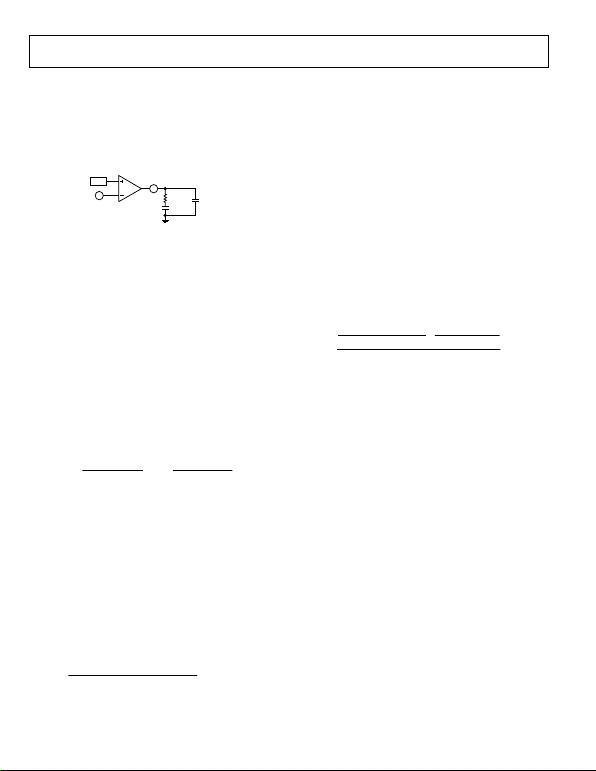Rev. C | Page 18 of 32
For low ESR output capacitors, such as ceramic capacitors, C2
is small, generally in the range of 10 pF to 400 pF. Because of the
parasitic inductance, resistance, and capacitance of the PCB layout,
the RCOMP, CCOMP, and C2 values might need to be adjusted by
stable operating system and achieve optimal transient performance.
For most applications, RCOMP is in the range of 5 kΩ to 100 kΩ,
and CCOMP is in the range of 100 pF to 30 nF.
COMP
C
COMP
R
COMP
C2
REF g
m
23
06090-030
Figure 31. Compensation Components
SLOPE COMPENSATION
compensation. Slope compensation is required by current-
mode regulators to stabilize the current-control loop when
operating in continuous conduction and the switching duty
cycle is greater than 50%.
Slope compensation is achieved by internally forcing a ramping
current source out of the CS current-sense pin. By placing a resistor
between the CS pin and the current sensing device (the drain of
the external MOSFET in the case of lossless current sensing or
the source of the MOSFET if a current-sense resistor is used), a
voltage is developed across the resistor that is proportional to
the slope-compensation current.
To ensure stability of the current-mode control loop, use a
compensation voltage slope that is equal to or greater than one-
half of the current-sense representation of the inductor current
downslope. Therefore, it follows that
L
VVV
R
ft
fI
RIND
OUT
CS
SWMINOFF,
SWSC,PK
S
1
2 (33)
where:
RS is the slope-compensation resistor.
ISC,PK is the peak slope-compensation current.
fSW is the switching frequency.
RCS is the current-sense resistor.
VOUT is the regulated output voltage.
VD is the forward-voltage drop of the diode.
VIN is the input voltage.
tOFF,MIN is the minimum off time.
L is the power-stage inductor. In the case of lossless current
sensing, RCS is equal to the on resistance, RDSON, of the external
power MOSFET. Otherwise, RCS represents the external current-
sense resistor.
Solving for RS gives the following slope-compensation criterion:
LfI
ftVVVR
R
SWPKSC
SWMINOFF
IND
OUT
CS
S
,
,
2
1 (34)
Keep in mind that the above inequality is a function of both
ADP1621 parameters and off-chip components, the values of
which vary from device to device and with temperature. Select
RS to ensure current-loop stability for all possible variations.
After accounting for parameter variations, use values of RS that
are as close to the calculated limit as possible because excessive
slope compensation reduces the benefits of current-mode control
and increases the “softness” of the current limit, as discussed in the
Current Limit section. Given a typical peak slope-compensation
current of 70 μA, RS must not exceed 1.6 kΩ because the voltage at
the CS pin is typically clamped at 116 mV. It is also recommended
that RS be greater than 20 Ω. If the calculated RS is greater than
1.6 kΩ, the parameters in Equation 34, such as RCS, fSW, and L,
can be adjusted such that RS is less than 1.6 kΩ.
In conclusion, the value of RS should be 20 Ω ≤ RS ≤ 1.6 kΩ.
CURRENT LIMIT
The current limit in the ADP1621 limits the peak inductor
current and is achieved by the COMP voltage clamp. The peak
inductor current, IL,PK, is given by
CS
SWMINOFF
SPKSCZCTCOMPCLAMPCOMP
PKL R
ft
DRI
n
VV
I
,
,,,
,
1 (35)
where:
VCOMP,CLAMP is the COMP clamp voltage (typically 2.0 V).
VCOMP,ZCT is the COMP zero-current threshold (typically 1.0 V).
n is the current-sense amplifier gain (typically 9.5).
ISC,PK is the peak slope-compensation current (typically 70 μA).
RS is the slope-compensation resistor.
D is the duty cycle.
fSW is the switching frequency.
tOFF,MIN is the minimum off time (typically 190 ns).
RCS is the current-sense resistor. In the case of lossless current
sensing, RCS is equal to the on resistance, RDSON, of the external
power MOSFET. Otherwise, RCS represents the external current-
sense resistor.
The current limit in the ADP1621 is a soft current limit. When
the inductor current reaches the IL,PK limit given in Equation 35,
the duty cycle decreases, and the output voltage drops below the
desired voltage. The IL,PK limit in Equation 35 then increases in
response to the smaller duty cycle, D. The larger the slope-
compensation resistor, RS, the larger the effect on IL,PK for an
incremental decrease in D. This behavior results in a soft current
limit for the ADP1621. Use values of RS that are as close as possible
to the calculated limit derived from Equation 34. If high-precision
current limiting is required, consider inserting a fuse in series
with the inductor.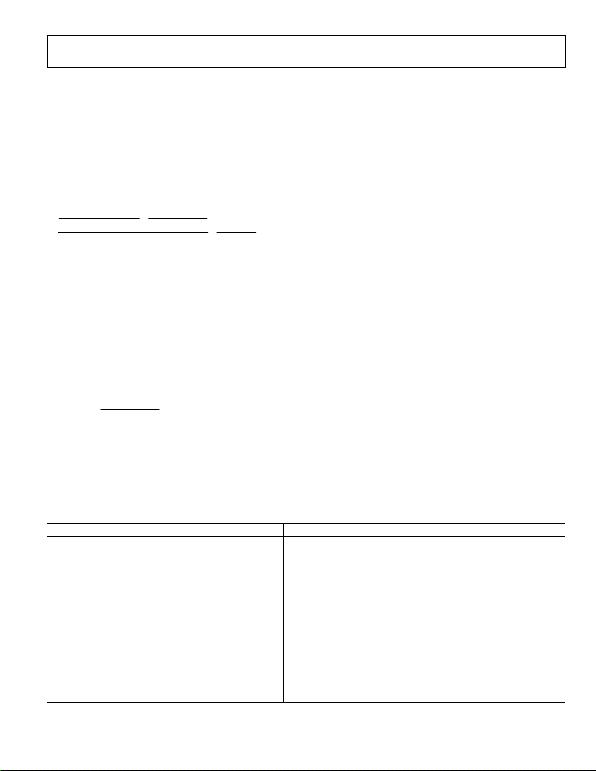Rev. C | Page 19 of 32
Also, keep in mind that the current limit is a function of both
ADP1621 parameters and off-chip components, the values of
which vary from device to device and with temperature. If
lossless current sensing is used, consider that the on resistance
of a MOSFET typically increases with increasing junction
temperature.
The peak inductor current limit also limits the maximum load
current at a given output voltage. The maximum load current,
assuming CCM operation, is given by

,
Lf DV
R
ft
DRI
n
VV
SW
IN
CS
SWMINOFF
SPKSCZCTCOMPCLAMPCOMP
2
1,
,,,
(36)
below the desired voltage.
Discontinuous Conduction Mode
With light loads, the average inductor current is small, and,
depending on the converter design, the instantaneous inductor
current may reach 0 during the time when the MOSFET is off.
This mode of operation is termed discontinuous conduction
mode. The condition for entering discontinuous conduction
mode in a boost converter is

SW
IN